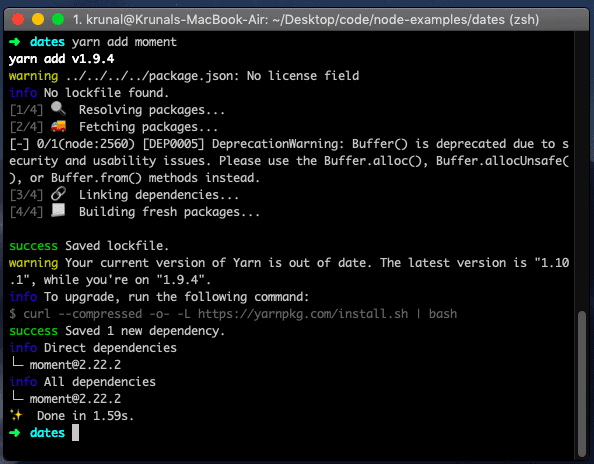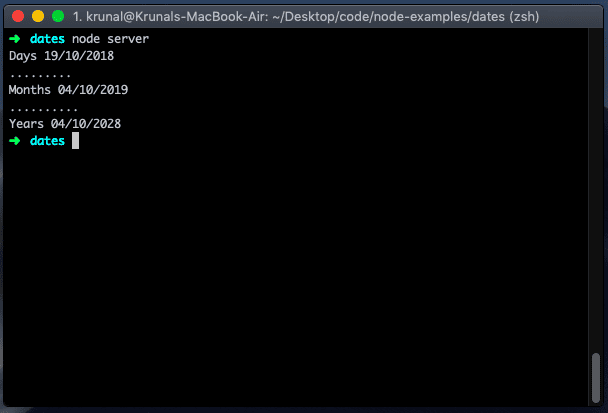# Moment JS Tutorial: The Complete Guide

The moment.js is an excellent library JavaScript library that helps you manage dates in the browser and Node.jsWorking with the dates and times has always been a bit difficult. So I have always thought the JavaScript library for manipulating dates would be beneficial.

Moment.js is an impressive JavaScript library for validating, parsing, and manipulating dates and times. The moment object in Moment.js is mutable. It means that operations like add, subtract, or set change the original moment object.## Moment JS

We will use Node.js for this example, as it can be helpful on a client(browser) and server(node.js).

## Step 1: Install Moment.js.

First, create one folder using the following command.

```mkdir dates
cd dates```

Okay, now create the package.json file using the following command.

`npm init -y`

Now, install the moment.js using the following command.

```npm install moment --save

# orNow, create one file called the server.js inside the root and add the following code.

```// server.js

const moment = require('moment');

console.log(moment().format('DD/MM/YYYY'));```

Save the file and go to the terminal and start the node.js server.

`node server`

You can see today’s date as formatted. The moment() gives the current date and time, while format() converts the current date and time to the specified format.

## #Get the current date and time at the moment.

You can write the following code.

```const moment = require('moment');

const date = moment();

console.log(date);```

## #Manipulating Dates

There are some options for manipulating a moment object. For example, you can add or subtract the days, months, years, etc. You can achieve this manipulation via the add() and subtract() methods.

```moment().add(Number, String);

Let us take the following example.

```// server.js

const moment = require('moment');

const days = moment().add(15, 'days').format('DD/MM/YYYY');    // adds 15 days to current date
const months = moment().add(12, 'months').format('DD/MM/YYYY');  // adds 12 months to current date
const years = moment().add(10, 'years').format('DD/MM/YYYY');   // adds 10 years to current dateconst date = moment();

console.log('Days', days);
console.log('.........');
console.log('Months', months);
console.log('..........');
console.log('Years', years);```

Save the file and run the node server.There are also some shorthand keys if you’re into that whole brevity thing.

Key Shorthand
years y
quarters Q
months M
weeks w
days d
hours h
minutes m
seconds s
milliseconds ms

If you need to add multiple keys simultaneously, you can pass them in as an object literal.

```moment().add(7, 'days').add(1, 'months'); // with chaining

### #Subtract Syntax

```moment().subtract(Number, String);
moment().subtract(Duration);
moment().subtract(Object);```

Let us take an example.

```// server.js

const moment = require('moment');

const days = moment().subtract(15, 'days').format('DD/MM/YYYY');    // subtracts 15 days to current date
const months = moment().subtract(12, 'months').format('DD/MM/YYYY');  // subtracts 12 months to current date
const years = moment().subtract(10, 'years').format('DD/MM/YYYY');   // subtracts 10 years to current dateconst date = moment();

console.log('Days', days);
console.log('.........');
console.log('Months', months);
console.log('..........');
console.log('Years', years);```

## Calculating the Difference Between Dates

Moment.js offers a way to compute the difference between two dates. The difference is calculated milliseconds by default, but can also be returned in days, months, years, etc. Call the diff() method on the moment to compute a difference. The diff() method takes a date as its first argument. After that, the unit of time can be specified using an optional second argument.

Let us take an example of the difference between dates.

```// server.js

const moment = require('moment');

const dateB = moment('2018-09-10');
const dateC = moment('1993-09-10');

console.log('Difference is ', dateB.diff(dateC, 'days'), 'days');
console.log('Difference is ', dateB.diff(dateC, 'months'), 'months');
console.log('Difference is ', dateB.diff(dateC, 'years'), 'years');```## #Date Comparison Methods

Moment.js also provides date comparison methods. These methods are isBefore()isAfter(), and isSame(). As the names suggest, these methods return Boolean values indicating if one date is before, after, or equal to another date.

Let us take an example.

```const moment = require('moment');

console.log(moment('2018-09-10').isAfter('2018-09-09')); // returns false
console.log(moment('2018-09-10').isAfter('2018-11-20')); // returns true```

## Time from now

The syntax is the following.

```moment().fromNow();
moment().fromNow(Boolean);```

The conventional way of displaying time is handled by `moment#fromNow`. It is sometimes called time ago or relative time.

Let us take an example.

```// server.js

const moment = require('moment');

console.log(moment([1993, 09, 10]).fromNow()); // 25 years ago```

## Parse as an Array

The syntax is the following.

`moment(Number[]);`

Let us take an example.

```// server.js

const moment = require('moment');

moment();        // January 1st
moment([1993, 10]);     // September 1st
moment([1993, 09, 10]); // September 10th```

## Moment Clone

The syntax is the following.

`moment(Moment);`

Let us take an example.

```// server.js

const moment = require('moment');

const a = moment();
const b = moment(a);
a.year(2018);
console.log(b.year()); // 1993```

You can find more about the moment’s official documentation.

That’s it.

This site uses Akismet to reduce spam. Learn how your comment data is processed.